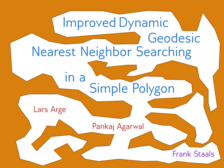Improved Dynamic Geodesic Nearest Neighbor Searching in a Simple Polgyon

AbstractWe present an efficient dynamic data structure that supports geodesic nearest neighbor queries for a set $$S$$ of point sites in a static simple polygon $$P$$. Our data structure allows us to insert a new site in $$S$$, delete a site from $$S$$, and ask for the site in $$S$$ closest to an arbitrary query point $$q \in P$$. All distances are measured using the geodesic distance, that is, the length of the shortest path that is completely contained in $$P$$. Our data structure achieves polylogarithmic update and query times, and uses $$O(n\log^3n\log m + m)$$ space, where $$n$$ is the number of sites in $$S$$ and $$m$$ is the number of vertices in $$P$$. The crucial ingredient in our data structure is an implicit representation of a vertical shallow cutting of the geodesic distance functions. We show that such an implicit representation exists, and that we can compute it efficiently.

Corresponding Publications

• Improved Dynamic Geodesic Nearest Neighbor Searching in a Simple Polgyon

Proc. 34th Annual Symposium on Computational Geometry, 2018
@inproceedings{dyn_geod_nn2018,
author = {Agarwal, Pankaj K. and Arge, Lars and Staals, Frank},
title = {Improved Dynamic Geodesic Nearest Neighbor Searching in a Simple Polgyon},
booktitle = {Proc. 34th Annual Symposium on Computational Geometry},
year = {2018},
editor = {Bettina Speckmann and Csaba D. T{\'o}th},
location = {Budapest, Hungary},
numpages = {14},
keywords = {data structure, simple polygon, geodesic distance
, nearest neighbor searching, shallow cutting},
category = {datastructures},
series = {Leibniz International Proceedings in Informatics (LIPIcs)},
publisher = {Schloss Dagstuhl--Leibniz-Zentrum fuer Informatik},
pages = {4:1--4:14},
isbn = {978-3-95977-066-8},
issn = {1868-8969},
volume = {99},
url = {https://doi.org/10.4230/LIPIcs.SoCG.2018.4},
doi = {10.4230/LIPIcs.SoCG.2018.4},
}